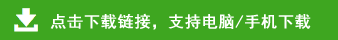# 小学四年级数学上册三位数乘两位数笔算练习题100道

三位数乘两位数笔算练习题

865×6=     612×12=     457×69=

862×68=    524×29=     541×9=

681×54=    683×40=     254×24=

260×23=    123×25=     724×6=

118×58=    148×42=     979×18=

84×33=     509×61=     728×31=